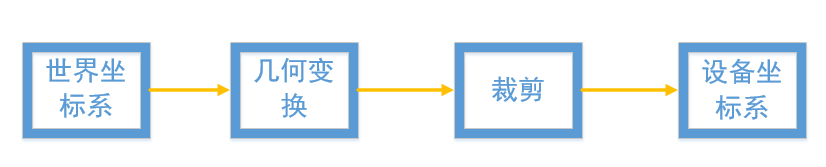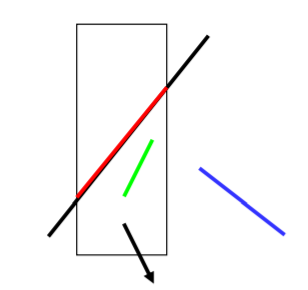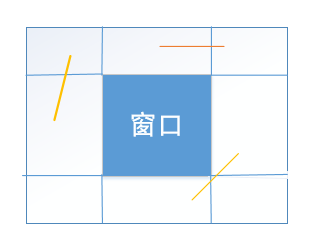﻿ 裁剪：编码裁剪算法

### 裁剪：编码裁剪算法## 裁剪的定义## 直线段的裁剪

1. 完全可见：两个端点都位于窗口内
2. 非显然不可见：线段至少有一端点位于窗口外
3. 显然不可见：两个端点都位于窗口外？## 编码算法

(x<x_{min}),(D_0=1)，否则为(0)

(x>x_{max}),(D_1=1)，否则为(0)

(y<y_{min}),(D_2=1)，否则为(0)

(y>y_{max}),(D_3=1)，否则为(0)

• (code_1|code_2=0),线段在窗口内，完全可见
• (code1&code_2 eq0),线段在窗口外，非完全可见